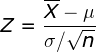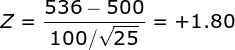# One-Sample Z Test Solution

### Example homework problem:

Twenty five high school students complete a preparation program for taking the SAT test.  Here are the SAT scores from the 25 students who completed the SAT prep program:

```434 694 457 534 720 400 484 478 610 641 425 636 454
514 563 370 499 640 501 625 612 471 598 509 531```

The mean of these scores is 536.00.  We know that the population average for SAT scores is 500 with a standard deviation of 100.

The question is, are these students’ SAT scores (M = 536) significantly greater than a population mean of 500 with a population standard deviation of 100? Note that the the maker of the SAT prep program claims that it will increase (and not decrease) your SAT score.  So, you would be justified in conducting a one-directional test. (alpha = .05).

The goal of your analysis is to test for a significant deviation between your sample mean and the population mean. In the case, the population standard deviation is a known parameter.

So, we will test the mean of these data against the value 500 with a one-sample Z test of the mean:The numerator of the formula is equal to your sample mean minus the population value you are comparing your sample to. The denominator is equal to standard error: the standard deviation of the population divided by the square root of n (the number of scores in your sample). If you would like help computing the sample mean, please see the documentation for the explore procedure.

Compute test statistic. You will find that the sample mean is equal to 536.00. Now, compute your Z statistic:Conduct hypothesis test. Since the company promoting the SAT prep program specifically claims that the program will improve SAT scores, we will conduct a directional (i.e., one-tailed) test with alpha = .05. When you check a table of Z values, you will find that the critical value of Z is 1.65. Since your obtained value of Z (+1.80) was greater than 1.65, you would conclude that the prep program resulted in a significant  improvement in SAT scores.

1.Haruna March 23, 2018 at 11:28 am

Good day,
I’d like to ask a question.
In this solution, i did not get the point where the sample mean is equal to 536.

•Bissonnette, Victor March 23, 2018 at 1:12 pm

Thanks for your question. I’m sorry for the confusion — this example was worded poorly. I’ve revised this example a bit to clarify. The mean of the 25 sample scores should be equal to 536.00. This is the sample mean being tested with the one-sample z test. Let me know if you have any more questions.

2.Hannah October 9, 2018 at 10:24 am

I have a question that is similar that I am confused on.
The Scholastic Aptitude Test (SAT) in which scores in the general population are approximately normally distributed with 500 ±100 (µ±σ). Suppose we select a sample of 25 high school students who complete a preparation program for taking the SAT test. In this sample, we record a mean equal to 536 (M=536). Are these students’ SAT scores (M = 536) significantly greater than a population mean of 500 with a population standard deviation of 100? Compute the one-sample z test (one-tailed test) to decide whether to retain or reject the null hypothesis at the .05 level of significance (α=.05). Steps 1-4

Can you explain to me what steps 1-4 would be?

•Bissonnette, Victor October 9, 2018 at 10:37 am

A formal null-hypothesis test typically includes these steps:
— specify the null and alternative hypotheses
— specify your test statistic and compute the value of your test statistic
— make a decision: reject or fail to reject the null hypothesis
— (in my class: state a conclusion describing the results of the study)

3.Robert Tata October 15, 2018 at 5:48 pm

Im curious on how you found the critical value

•Bissonnette, Victor October 16, 2018 at 8:18 am

Since we wanted to conduct a one-tailed hypothesis test with alpha =.05, you would find the critical value by looking in a normal / z table (https://sites.berry.edu/vbissonnette/wp-content/uploads/sites/21/2015/07/z.pdf) and look up the value of z for a tail probability as close to .05 as you can. This will result in finding a z of 1.65.

4.jennie camposano May 18, 2019 at 2:06 am

how you’ll find the null and alternative hypothesis?

•Bissonnette, Victor May 20, 2019 at 7:26 am

The alternative hypothesis is a statement that there is a significant treatment effect present. In this case, the alternative hypothesis would be that the SAT prep program caused the SAT scores to be greater than the population mean (500) in the students who completed the program.

The null hypothesis is a statement that there was no treatment effect. In this case, the null hypothesis would be that the SAT prep program had no effect on SAT scores (i.e., equal to 500) or, caused the SAT scores to be lower than 500.